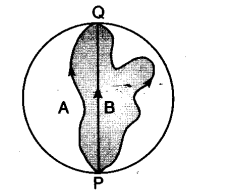# Three girls skating on a circular ice ground of radius 200 m start from a point P on the edge of the ground and reach a point Q diametrically opposite to P following different paths as shown in Figure,

Three girls skating on a circular ice ground of radius 200 m start from a point P on the edge of the ground and reach a point Q diametrically opposite to P following different paths as shown in Figure, (i) What is the magnitude of the displacement vector for each ? (ii) For which girl is this equal to the actual length of path skate ?(i) PQ = diameter
= displacement for each girl = 2r = 2 x 200 = 400 m
Since, displacement vector does not depend upon the actual path length and it is the shortest distance between initial and final position, so in the case of each girl the displacement is 400 m.

1 Like